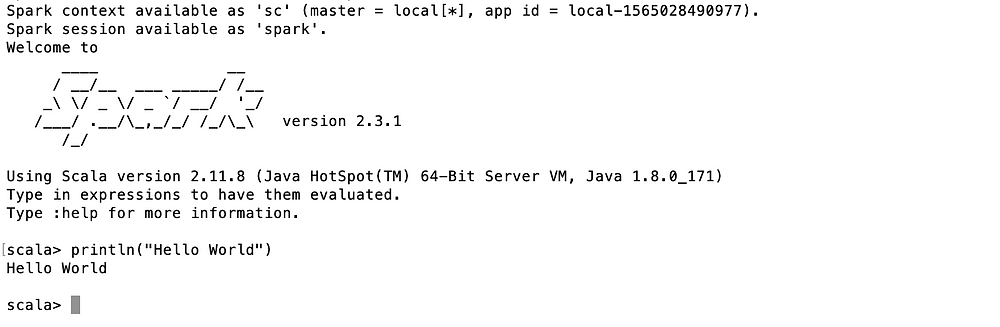# Just enough Scala for Spark

Updated: Oct 25, 2019

In this tutorial you will learn just enough Scala for Spark, it's like a quick guide for Scala basics needed for Spark programming, Scala syntax and few Scala examples.

Well, you can't become Scala expert in a day but after reading this post you will be able to write Spark programs. I will be using Spark-shell to run Scala commands, so no installation needed if you have Spark shell running on your machine. I would encourage you to run these commands side-by-side on your machine.

Staring with printing "Hello World", for example,scala> println("Hello World")

Hello World

For comments you can use double forward slash, or for multiline comments you can use similar syntax like Java. For example, ignore the pipe character it's because I am using spark-shell.

scala> // Hello Data Nebulae - This is single line comment

scala> /* Hello World

| This is multi-line comment

| Data Nebulae

| */

We have two types of variables in Scala - mutable and immutable variables. Mutable variable are defined with var keyword and immutable variable with val keyword.

You can't re-assign immutable variables. For example,

scala> val myNumber :Int = 7

myNumber: Int = 7

scala> var myWord :String = "Hello"

myWord: String = Hello

Because myNumber is immutable variable so re-assignment failed

scala> myNumber = 10

<console>:25: error: reassignment to val

myNumber = 10

scala> myWord = "Dataneb"

myWord: String = Dataneb

You can specify datatype (Int, Double, Boolean, String) in front of variable name, if not Scala compiler will automatically assign the type (called variable type inference).

scala> val myNumber :Int = 10

myNumber: Int = 10

scala> val myFlag = true

myFlag: Boolean = true

You can also assign variables in pairs, basically tuples similar to Python,

scala> val (x, y) = (1, 5)

x: Int = 1

y: Int = 5

keep going..

scala> var (x, y, z) = (1, 2, "Hello")

x: Int = 1

y: Int = 2

z: String = Hello

You can pass these variables to println function

scala> println (x)

1

String interpolation, like you do in other languages s with double quotes;

scala> println(s"Value of x is: \$x")

Value of x is: 1

Similar to other languages, you can create a range with step-size and print for each element.

scala> (1 to 5).foreach(println)

1

2

3

4

5

scala> (5 to 1 by -1)

res144: scala.collection.immutable.Range = Range(5, 4, 3, 2, 1)

scala> (5 to 1 by -2)

res145: scala.collection.immutable.Range = Range(5, 3, 1)

Strings are surrounded by double quotes and characters with single quotes, for example,

scala> "Hello Word"

res111: String = Hello Word

scala> 'H'

res112: Char = H

scala> :type ('H')

Char

You can apply similar methods like other languages, length, substring, replace etc, for example

scala> "Hello World".length

res113: Int = 11

scala> "Hello World".size

res1: Int = 11

scala> "Hello World".toUpperCase

res2: String = HELLO WORLD

scala> "Hello World".contains('H')

res5: Boolean = true

scala> 19.toHexString

res4: String = 13

scala> "Hello World".take(3)

res114: String = Hel

scala> "Hello World".drop(3)

res115: String = lo World

scala> "Hello World".substring(3,6)

res116: String = "lo "

scala> "Hello World".replace("H","3")

res123: String = 3ello World

scala> "Hello".map(x=>(x,1))

res7: scala.collection.immutable.IndexedSeq[(Char, Int)] = Vector((H,1), (e,1), (l,1), (l,1), (o,1))

Array, List, Map, Set - behaves similarly like other languages data structures

scala> val a = Array("Hello", "World", "Scala", "Spark")

a: Array[String] = Array(Hello, World, Scala, Spark)

// you can access the elements with index positions

scala> a(0)

res159: String = Hello

scala> (a(0),a(3))

res160: (String, String) = (Hello,Spark)

Similarly List..

// List of Integers

scala> val l = List(1, 2, 3, 4, 5)

l: List[Int] = List(1, 2, 3, 4, 5)

// List of strings

scala> val strings = List("Hello", "World", "Dataneb", "Spark")

strings: List[String] = List(Hello, World, Dataneb, Spark)

// List of List

scala> val listOfList = List(List(1,2,3), List(2,6,7), List(2,5,3))

listOfList: List[List[Int]] = List(List(1, 2, 3), List(2, 6, 7), List(2, 5, 3))

scala> val emptyList = List()

emptyList: List[Nothing] = List()

Similarly Map..

scala> val m = Map("one" -> 1, "two" -> 2 )

m: scala.collection.immutable.Map[String,Int] = Map(one -> 1, two -> 2)

scala> m("two")

res163: Int = 2

Set, returns boolean

scala> val s = Set("Apple", "Orange", "Banana")

s: scala.collection.immutable.Set[String] = Set(Apple, Orange, Banana)

scala> s("Apple")

res164: Boolean = true

scala> s("Grapes")

res165: Boolean = false

Arithmetic operations + (adds), -(subtracts), *(multiply), / (divide), %(remainder) for example,

scala> val (x, y) = (5, 8)

x: Int = 5

y: Int = 8

scala> y%x

res95: Int = 3

scala> res95 + 7

res110: Int = 10

scala> "Hello" + " World"

res0: String = Hello World

Relational operators ==, !=, <, >, >=, <= for example,

scala> y > x

res96: Boolean = true

Logical operators &&, ||, ! for example,

scala> !(y>x && x>y)

res98: Boolean = true

Assignment operators =, +=, %= etc for example, like other languages x+=y is same as x=x+y;

scala> var (x, y) = (5, 8)

x: Int = 5

y: Int = 8

scala> x+=y

scala> x

res102: Int = 13

Array of integers, with println and index

scala> val a = Array(1, 2, 3)

a: Array[Int] = Array(1, 2, 3)

scala> println(s"Sum is \${a(0) + a(1) + a(2)}")

Sum is 6

Defining function has also similar syntax (ignore | character), (Int, Int) => (Int, Int) means function takes two integer argument and returns two integers.

scala> def squareOfNumbers(x: Int, y: Int): (Int,Int) = {(x*x, y*y)

| // for multiline you have to use curly {} brackets

| }

squareOfNumbers: (x: Int, y: Int)(Int, Int)

scala> squareOfNumbers(2,3)

res131: (Int, Int) = (4,9)

Lambda function, if you will not mention datatype, Scala compiler will automatically decide it (inference).

scala> (x:Int) => x+x

res132: Int => Int = <function1>

Int => Int means function takes integer return integer

scala> val func: Int => Int = x => x + x